# 吴恩达深度学习学习笔记——C1W4——深层神经网络——作业1——从零开始搭建一个深度神经网络

01/27 08:58

https://github.com/pandenghuang/Andrew-Ng-Deep-Learning-notes/tree/master/assignments/C1W4

# Building your Deep Neural Network: Step by Step

Welcome to your week 4 assignment (part 1 of 2)! You have previously trained a 2-layer Neural Network (with a single hidden layer). This week, you will build a deep neural network, with as many layers as you want!

• In this notebook, you will implement all the functions required to build a deep neural network.
• In the next assignment, you will use these functions to build a deep neural network for image classification.

After this assignment you will be able to:

• Use non-linear units like ReLU to improve your model
• Build a deeper neural network (with more than 1 hidden layer)
• Implement an easy-to-use neural network class

...

## 1 - Packages

Let's first import all the packages that you will need during this assignment.

• numpy is the main package for scientific computing with Python.
• matplotlib is a library to plot graphs in Python.
• dnn_utils provides some necessary functions for this notebook.
• testCases provides some test cases to assess the correctness of your functions
• np.random.seed(1) is used to keep all the random function calls consistent. It will help us grade your work. Please don't change the seed.

...

## 2 - Outline of the Assignment

To build your neural network, you will be implementing several "helper functions". These helper functions will be used in the next assignment to build a two-layer neural network and an L-layer neural network. Each small helper function you will implement will have detailed instructions that will walk you through the necessary steps. Here is an outline of this assignment, you will:

• Initialize the parameters for a two-layer network and for an 𝐿L-layer neural network.
• Implement the forward propagation module (shown in purple in the figure below).
• Complete the LINEAR part of a layer's forward propagation step (resulting in 𝑍[𝑙]Z[l]).
• We give you the ACTIVATION function (relu/sigmoid).
• Combine the previous two steps into a new [LINEAR->ACTIVATION] forward function.
• Stack the [LINEAR->RELU] forward function L-1 time (for layers 1 through L-1) and add a [LINEAR->SIGMOID] at the end (for the final layer 𝐿L). This gives you a new L_model_forward function.
• Compute the loss.
• Implement the backward propagation module (denoted in red in the figure below).
• Complete the LINEAR part of a layer's backward propagation step.
• We give you the gradient of the ACTIVATE function (relu_backward/sigmoid_backward)
• Combine the previous two steps into a new [LINEAR->ACTIVATION] backward function.
• Stack [LINEAR->RELU] backward L-1 times and add [LINEAR->SIGMOID] backward in a new L_model_backward function
• Finally update the parameters.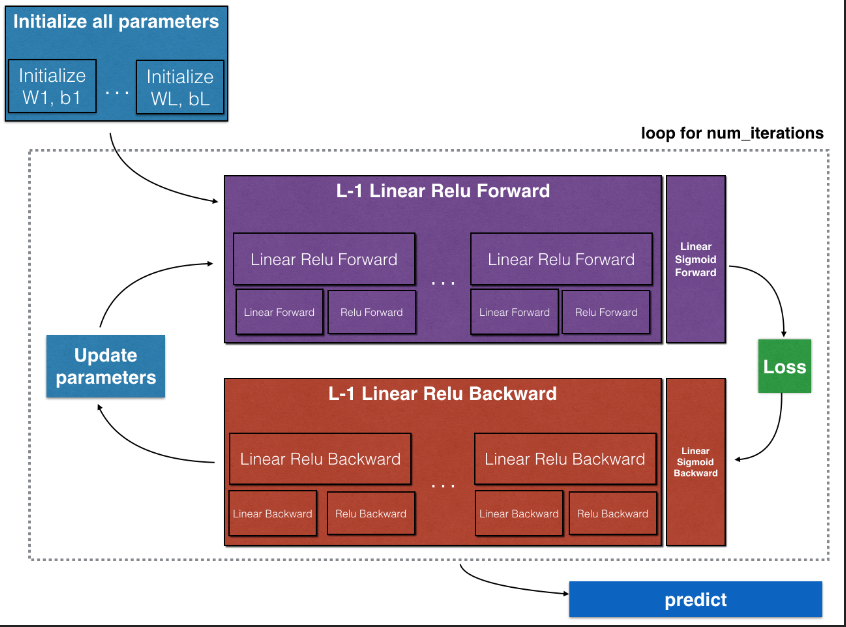...

## 3 - Initialization

You will write two helper functions that will initialize the parameters for your model. The first function will be used to initialize parameters for a two layer model. The second one will generalize this initialization process to 𝐿L layers.

### 3.1 - 2-layer Neural Network （2层神经网络的初始化）

Exercise: Create and initialize the parameters of the 2-layer neural network.

Instructions:

• The model's structure is: LINEAR -> RELU -> LINEAR -> SIGMOID.
• Use random initialization for the weight matrices. Use np.random.randn(shape)*0.01 with the correct shape.
• Use zero initialization for the biases. Use np.zeros(shape).

...

### 3.2 - L-layer Neural Network （任意层神经网络的初始化）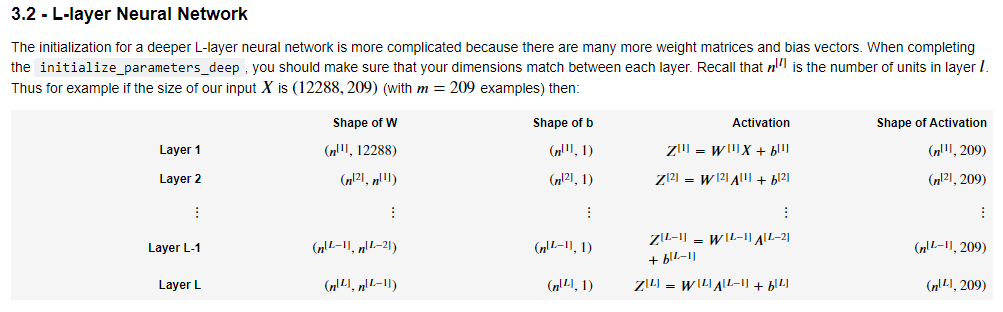...

## 4 - Forward propagation module

### 4.1 - Linear Forward（线性前向传播）

Now that you have initialized your parameters, you will do the forward propagation module. You will start by implementing some basic functions that you will use later when implementing the model. You will complete three functions in this order:

• LINEAR
• LINEAR -> ACTIVATION where ACTIVATION will be either ReLU or Sigmoid.
• [LINEAR -> RELU] ×× (L-1) -> LINEAR -> SIGMOID (whole model)

...

### 4.2 - Linear-Activation Forward （线性+可选激活函数前向传播）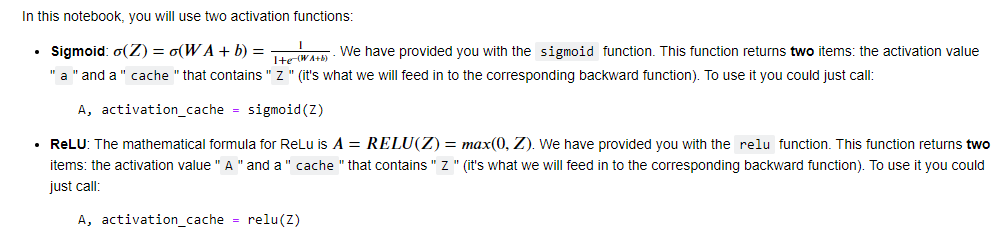### d) L-Layer Model （任意层模型）

For even more convenience when implementing the 𝐿L-layer Neural Net, you will need a function that replicates the previous one (linear_activation_forward with RELU) 𝐿−1L−1 times, then follows that with one linear_activation_forward with SIGMOID.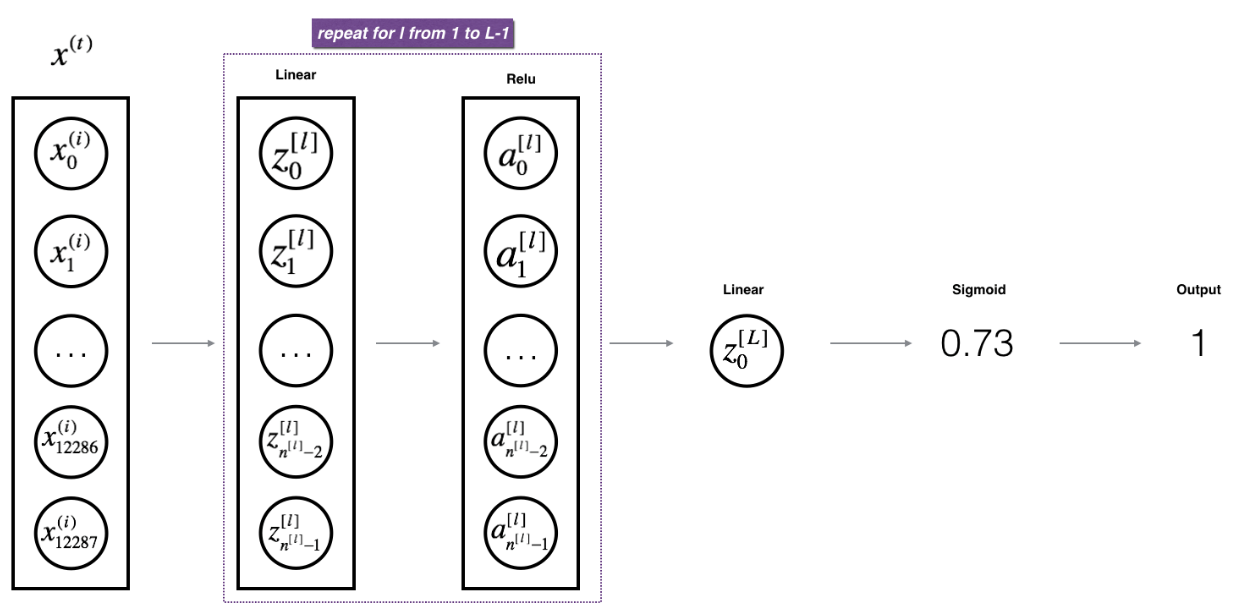...

## 5 - Cost function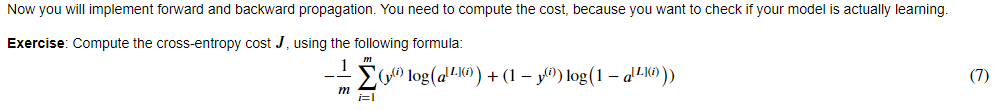...

## 6 - Backward propagation module

Just like with forward propagation, you will implement helper functions for backpropagation. Remember that back propagation is used to calculate the gradient of the loss function with respect to the parameters....

### 6.1 - Linear backward （线性反向传播）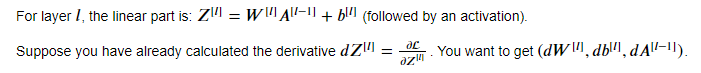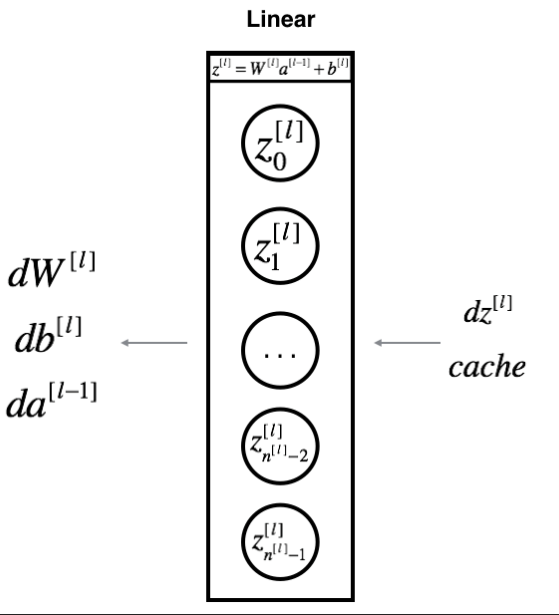...

### 6.2 - Linear-Activation backward （线性+激活函数反向传播）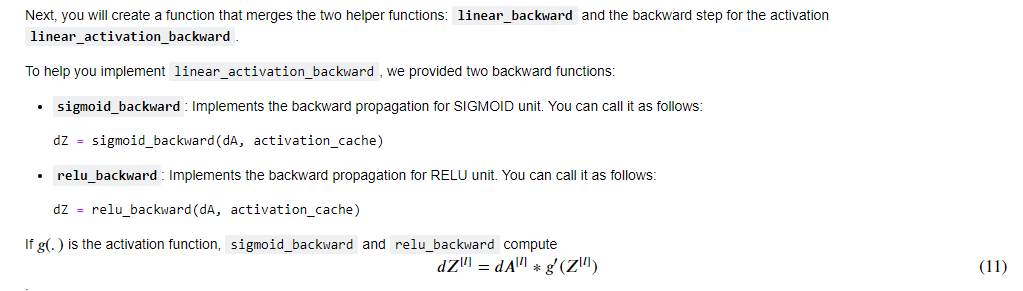...

### 6.3 - L-Model Backward（任意层反向传播）

Now you will implement the backward function for the whole network. Recall that when you implemented the L_model_forward function, at each iteration, you stored a cache which contains (X,W,b, and z). In the back propagation module, you will use those variables to compute the gradients. Therefore, in the L_model_backward function, you will iterate through all the hidden layers backward, starting from layer 𝐿L. On each step, you will use the cached values for layer 𝑙l to backpropagate through layer 𝑙l. Figure 5 below shows the backward pass.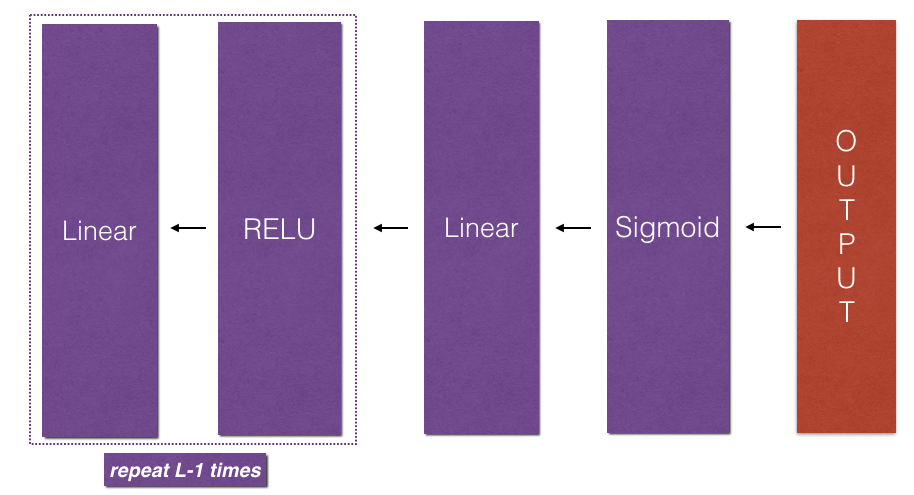...

### 6.4 - Update Parameters （更新参数）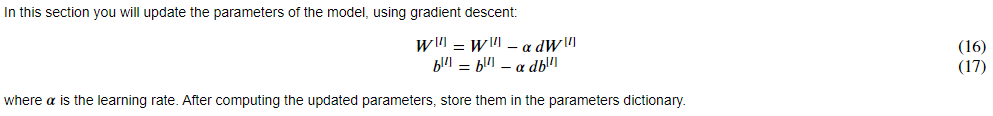...

## 7 - Conclusion （小结）

Congrats on implementing all the functions required for building a deep neural network!

We know it was a long assignment but going forward it will only get better. The next part of the assignment is easier.

In the next assignment you will put all these together to build two models:

• A two-layer neural network
• An L-layer neural network

You will in fact use these models to classify cat vs non-cat images!

0
0 收藏

### 作者的其它热门文章0 评论
0 收藏
0